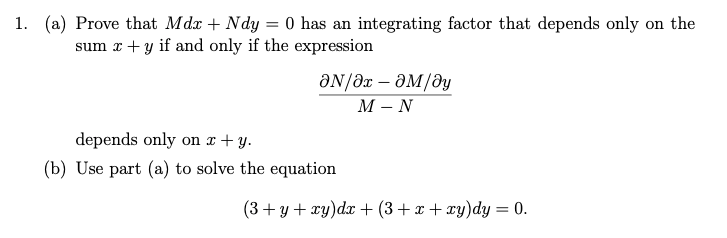# Question Solved1 Answer1. (a) Prove that Mdx + Ndy = 0 has an integrating factor that depends only on the sum x +y if and only if the expression an/ar - May M -N depends only on 2+y. (b) Use part (a) to solve the equation (3+ y + xy)dx + (3++xy)dy = 0.Transcribed Image Text: 1. (a) Prove that Mdx + Ndy = 0 has an integrating factor that depends only on the sum x +y if and only if the expression an/ar - May M -N depends only on 2+y. (b) Use part (a) to solve the equation (3+ y + xy)dx + (3++xy)dy = 0.
More
Transcribed Image Text: 1. (a) Prove that Mdx + Ndy = 0 has an integrating factor that depends only on the sum x +y if and only if the expression an/ar - May M -N depends only on 2+y. (b) Use part (a) to solve the equation (3+ y + xy)dx + (3++xy)dy = 0.